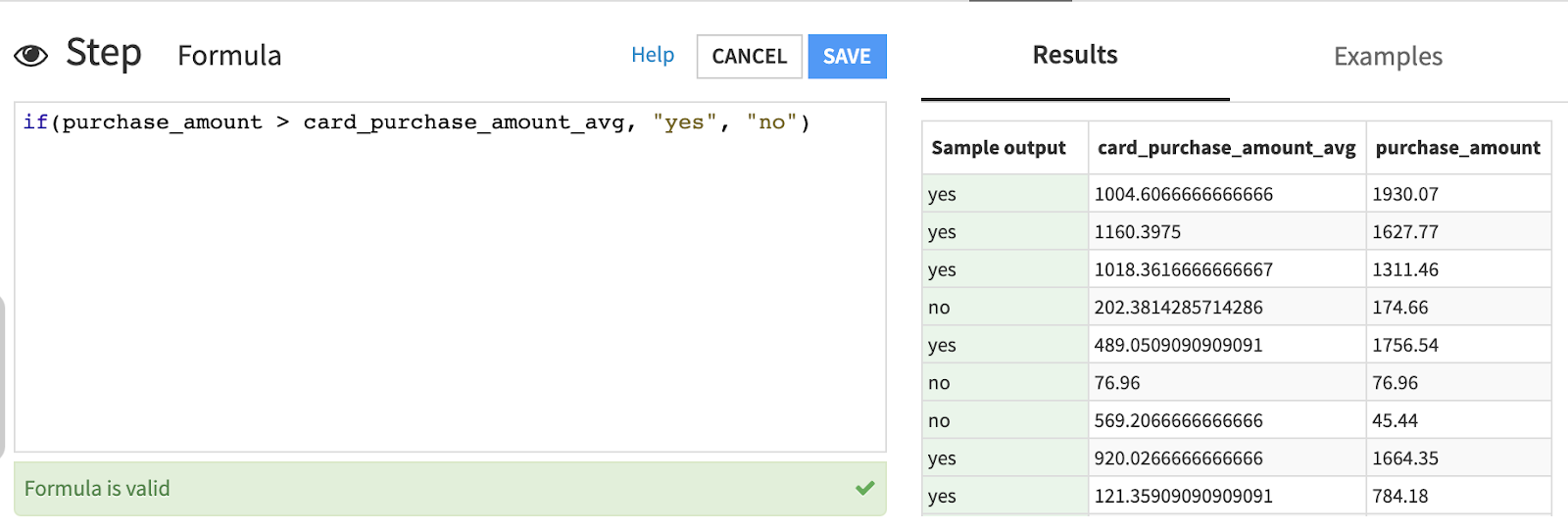The Prepare recipe in Dataiku contains 100+ processors for data preparation tasks. We’ll look at one of these functions: Dataiku formulas.

## Formulas¶

Dataiku has its own Formula language, similar to what we might find in a spreadsheet tool like Excel.

In a Prepare recipe, you can use a formula to create new columns or flag rows. Dataiku applies the Formula row by row. For each row, Dataiku returns the result and stores it in a new column.Here are some examples of useful Dataiku formula functions:

• The length() function returns the length of a string. This is useful when we want to filter rows based on the length of our text data.

• With a substring() function, we can extract a substring from a text column, by indicating the starting and ending position of the part that we want to extract.

• The rand() function generates random integers between the minimum and maximum values we choose. This is useful for generating simulation data.

• strval() allows us to access the values of columns, where the column name includes a space. Without the proper syntax, Dataiku displays a syntax error. To avoid this error, we wrap the column name in double quotations, and apply the “strval()” function. The value is returned as a string.

• Likewise, numval() evaluates the column name with spaces and returns the column value as a number.

### If statement¶

One of the most frequently used formula functions is the if() statement.

The if() statement takes three arguments:

• a condition that evaluates to true or false,

• a value returned when the condition is true, and

• the value returned when the condition is false.For example, let’s say we want to create a column that takes a value of either “0” or “1”. We want a “1” if the value in a certain column is greater than 100, if not, we want a “0”.

To accomplish this, we would write the following formula:

• if(column_name > 100, 1, 0)

#### Example: Flag large credit card purchases¶

To use an example, let’s say we have a credit card transaction dataset where we want to compare the amount of a given transaction to the average purchase amount for the credit card it was made with.

This could be useful for fraud detection. For example, If a card holder makes a disproportionately expensive purchase compared to the regular use of their credit card, we could flag the purchase as potentially fraudulent.

We first add a Formula step to create a new column named higher_than_avg_purchase. We then open the formula editor and write a formula expression to check whether the value of the purchase_amount column is higher than that of the card_purchase_amount_average column.

We’ll use the following formula expression:

• if(purchase_amount > card_purchase_amount_avg, "yes", "no")Once we click Save, Dataiku displays the newly created column. Each transaction is flagged as being more expensive than the card average or not.

We saw how we can leverage the Dataiku formula language to create new columns or flag rows using the Formula processor in the Prepare recipe. We can also use a formula in other visual recipes in Dataiku in the Computed Columns step.

## What’s next?¶

Now that you’ve learned more about two commonly used processors, you can visit the Academy to learn about other visual recipes, browse the Knowledge Base, or visit the Dataiku Processors reference.